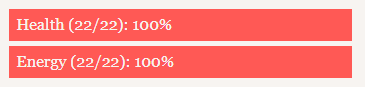# Increasing the value of variables in stat chart

Hello, I’m new to ChoiceScript and coding in general and I would appreciate any help I can get.

I was wondering what would be the correct code to increase the value of stat variables in stat percent charts?

For example, let’s say we have a Strength attribute which is displayed as

``````*stat_chart
percent strength Strength
percent charisma Charisma
percent intelligence Intelligence
``````

How do I set the max value for Strength to be 1000 instead of 100, so if it goes over or equal to 1000, the percent bar will be shown as 100% but if you have a value of 100 then it will be displayed as 10% of the percent bar?

You can use fairmath to add and subtract % to a variable. here is a guide to that.

As for changing the value of 100 to 1000 I think there is a way but I can’t find it right now.

Well, I think you can manually make the operation and update the value accordingly to the 0-100 scale
but if you want to display from 0-100 I don’t see why you need to go to 1000 if the bar only goes to 100.

Fair math will keep the value of the variable strictly within the percentile range, that is between 0 and 100.

To turn any value into a percentage, just divide it by its maximum.

``````*stat_chart
percent round(strength/1000*100) Strength
``````

There are definitely benefits, as you can have a wider range, you can use a different scale to grant points. Also, not everyone (and I mean me) likes fairmath, because change is exponential instead of linear it is much harder to balance the game properly without a lot of trial and error.

2 Likes

Basically, what you want is decimal precision.

I would suggest keeping double variables, e.g. `strength_real` and `strength_display`, and then updating them in a subroutine that checks whether they’ve gone over or under the limit. Something like this:

``````*label add_strength
*if ((sum >= 0) and (sum <= 1000))
*set strength_real sum
*set strength_display sum / 10
*return
``````

And then you would call it like `*gosub add_strength 5` or similar. Although I kept my universal subroutines in a separate file, so I used `*gosub_scene`.

1 Like

An example from my WIP, using the player’s health and energy:

``````*set rounded_health round((p_health / p_max_health) * 100)
*set rounded_energy round((p_energy / p_max_energy) * 100)
*stat_chart
percent rounded_health Health (\${p_health}/\${p_max_health})
percent rounded_energy Energy (\${p_energy}/\${p_max_energy})
``````

The result is shown like this:3 Likes